GeeksforGeeks App
Open AppBrowser
Continue

# Visualizing Solid Shapes

Any plane or any shape has two measurements like length and width, that is why it is called a two-dimensional(2D) object. Circle, square, triangles, rectangles, trapeziums, etc. are 2-D shapes. If an object has length, width and breadth then it is a three-dimensional object(3D). cube, pyramids, spheres, cylinders, cuboids are 3-D shapes. Any kind of solid shape occupies some space. A solid shape or figure is bounded by one or more surfaces. If any two faces of 3-D shapes meet together, we get a line segment which is called edge when more than two faces of the solid meet at one point then that point is called the vertex of solid. A 3-D solid have different views from different positions. A two-dimensional shape and three-dimensional shape can be named 2D and 3D objects.

Now, you can see some 2D and 3D objects are given below: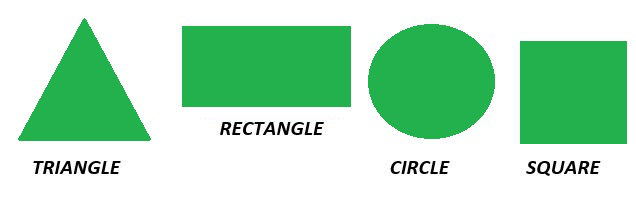2-D Shapes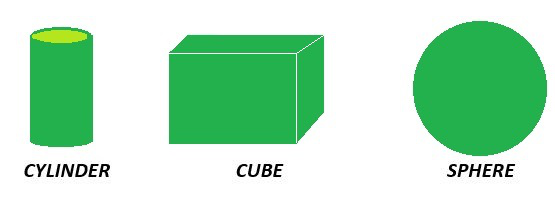3D -Shapes

### Faces, Edges, and Vertices

Face

A face refers to any single flat surface of a solid object. Solid shapes can have more than one face. The polygonal regions which a solid is made of are called faces.

Edges

An edge is a line segment on the boundary joining one vertex (corner point) to another. They serve as the junction of two faces. The faces meet at edges which are lines.

Vertices

A point where two or more lines meet is called a vertex. It is a corner. The point of intersection of edges denotes the vertices. These edges meet at vertices which are points

Now, if we talk about three-dimensional shapes then they have different numbers of faces, vertices, and edges. All that flat surface of shape is called a face. This flat shape is two-dimensional. The line segment where the faces of three-dimensional shapes meet each other is called the edge of the figure. The points or the corners where edges meet each other are called vertices.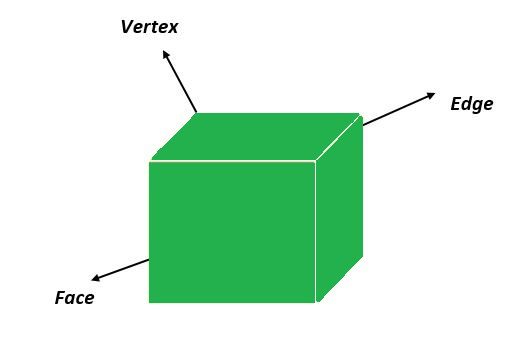Now, if we classified the number of faces, vertex and edge then this cuboid has 6 faces, 12 edges, and 8 vertices.

### View of 3D Shapes

Any three-dimensional figure or shape has a top view, side view, and front view.

Top View: Shape of the object when you see the object from the top or from directly above called as Top view of an object.

Side View: Shape of the object when you see the object from one side as mentioned in the below figure.

Front View: Shape of the object when you see the object from the front direction as mentioned in the below figure.

Now look at the cube, we will look at the top view, side view, and front view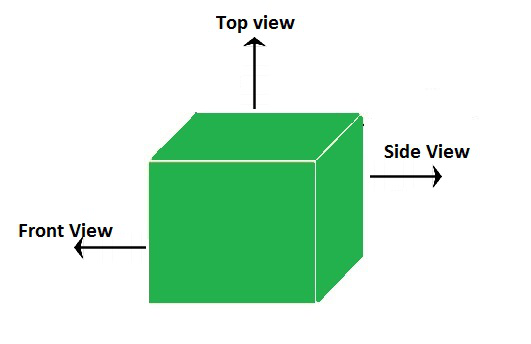Front View: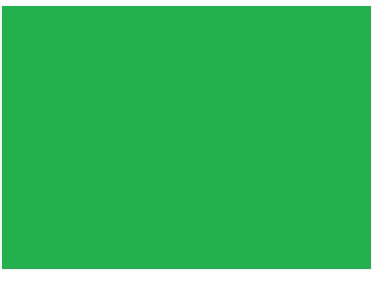All we see a front face which is a square.

Top View:All we see a top view which is also a square.

Side View:This is the side view (left and right) of the cube which also a square.

Note: A Cube will always look like a square whether its front view, side view or top view.

### Cylinder

A cylinder is a three-dimensional solid that contains two parallel bases connected by a curved surface. The bases are usually circular in shape. The perpendicular distance between the bases is denoted as the height “h” of the cylinder and “r” is the radius of the cylinder.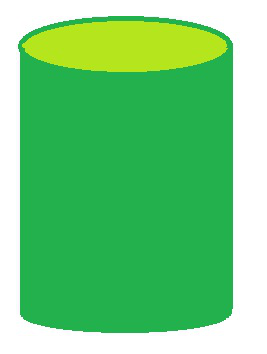Top view: When we see the cylinder from the top then it looks like a circle.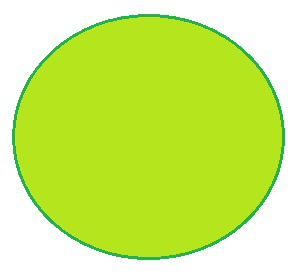Circle

Front View: When we see the cylinder from the front view then its looks like a rectangle.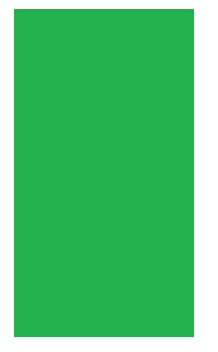Front View

Side View: When we see the cylinder from the side view then its looks like a rectangle.Side View

### Square Pyramid

A pyramid is a 3-dimensional geometric shape formed by connecting all the corners of a polygon to a central apex.

There are many types of pyramids. Most often, they are named after the type of base they have. Following is a square pyramid because of its base as a square.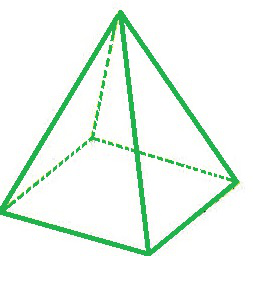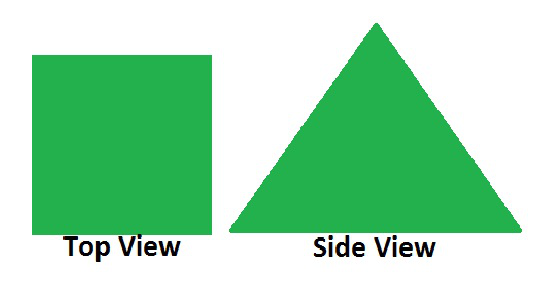Side view of pyramid will look like a triangular shape for left and right side.

Bottom of the pyramid has square shape.

Faces = 5

Edges = 8

Vertices = 5

### Triangular Pyramid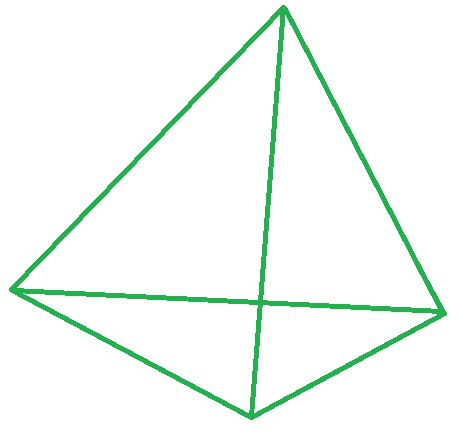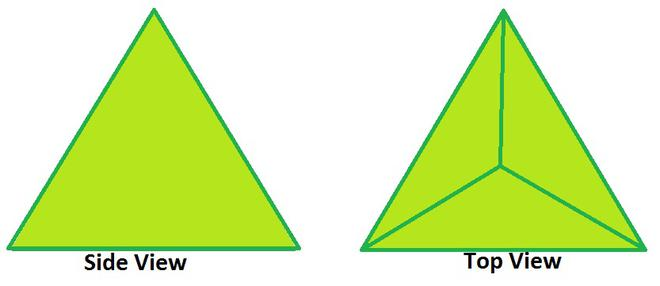Side view of the pyramid will look like a triangular shape for the left and right sides.

Bottom of the pyramid has a triangle shape.

Faces = 4

Edges = 6

Vertices = 4

### Cone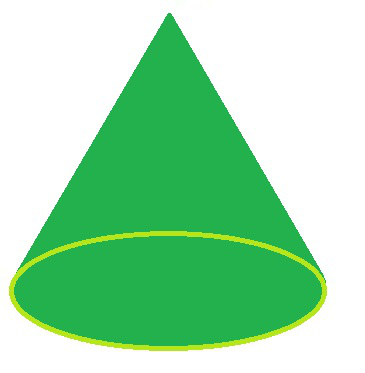Cone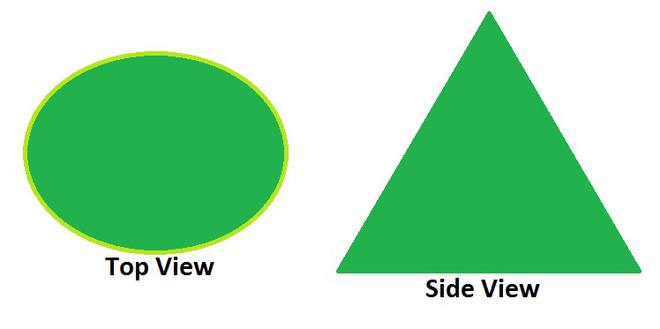Side view for cone, it will look like a triangle.

From the top, it will look like a circle.

Faces = 2

Edges = 2

Vertices = 1

### Sample Questions

Question 1: How many vertices are there in a sphere?

Sphere has no vertices in there because it has round shape.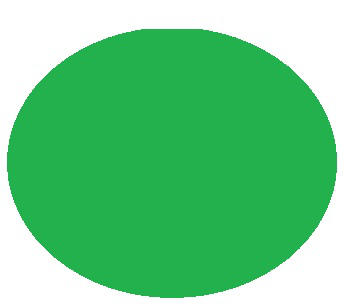Sphere

Question 2: Is a cone polyhedron? Give explanation.

Cone is not a polyhedron because it has round shape. Polyhedron is a 3D shape made by joining many polygons together. Polyhedron comes from Greek, poly means many and hadron means surfaces for example prism, cube, pyramid etc. are polyhedron 3D shapes.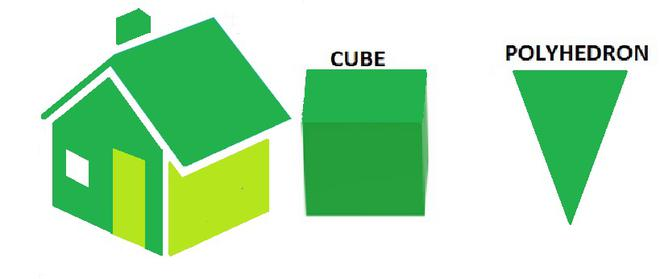Question 3: What is a triangular pyramid? What is a pyramid called if it has a square base?